# 【论文速览】CVPR2020 | CDVD-TSP: 基于时序清晰先验的级联深度视频去模糊模型

Posted by Haoran Bai on May 04, 2020 · 2 mins read

# CDVD-TSP: 基于时序清晰先验的级联深度视频去模糊模型

## 3. 算法详细介绍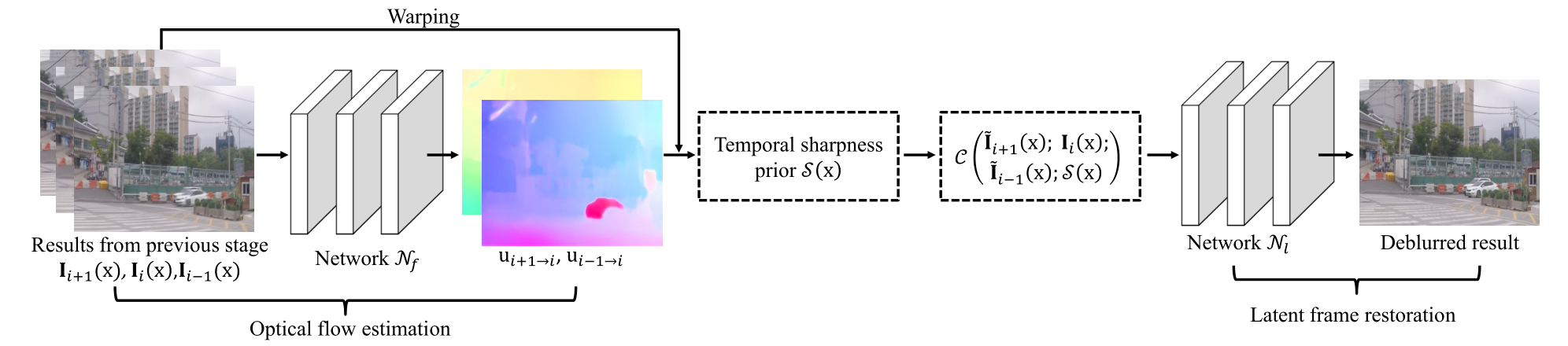### 3.1 光流估计模块

$u_{i\rightarrow i+1}=N_f(I_i;I_{i+1}) \tag{1}$

### 3.2 潜在清晰图像重建模块

$I_i(x)\leftarrow \frac{1}{3}(I_{i+1}(x+u_{i+1\rightarrow i})+I_{i-1}(x+u_{i-1\rightarrow i})+I_i(x)) \tag{2}$

$I_i(x)\leftarrow N_l(C(I_{i+1}(x+u_{i+1\rightarrow i});I_{i-1}(x+u_{i-1\rightarrow i});I_i(x))) \tag{3}$

### 3.3 时序清晰先验

$S_i(x) = exp(-\frac{1}{2} \sum_{j\&j\neq0}D(I_{i+j}(x+u_{i+j \rightarrow i}); I_i(x))) \tag{4}$

$D(I_{i+j}(x+u_{i+j \rightarrow i}); I_i(x)) = \sum_{y\in \omega(x)} \| I_{i+j}(y+u_{i+j \rightarrow i}) - I_i(y) \|^2 \tag{5}$

$I_i(x)\leftarrow N_l(C(I_{i+1}(x+u_{i+1\rightarrow i});I_{i-1}(x+u_{i-1\rightarrow i});I_i(x);S_i(x))) \tag{6}$

### 3.4 级联训练策略

$J(\theta_t)=\sum_{n=1}^N \sum_{i=1}^M \| F_{\theta_t}(B_{i-j}^n;...;B_i^n;...;B_{i+j}^n)-I_{gt,i}^n \|_1 \tag{7}$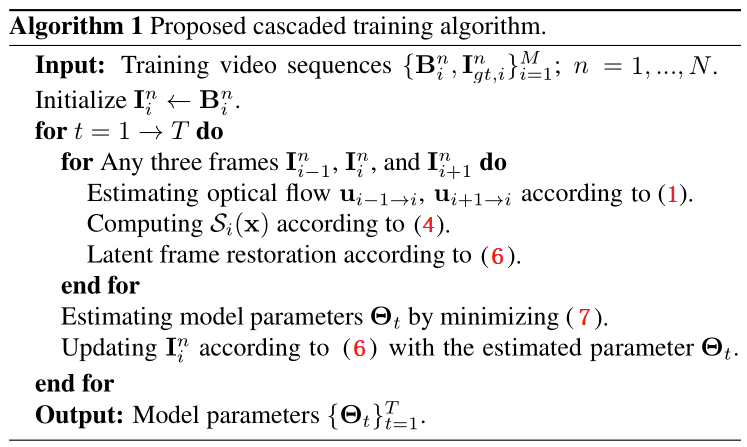## 4. 主要实验结果

### 4.1 基准数据集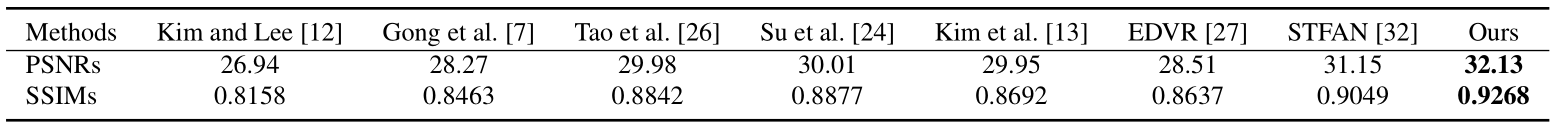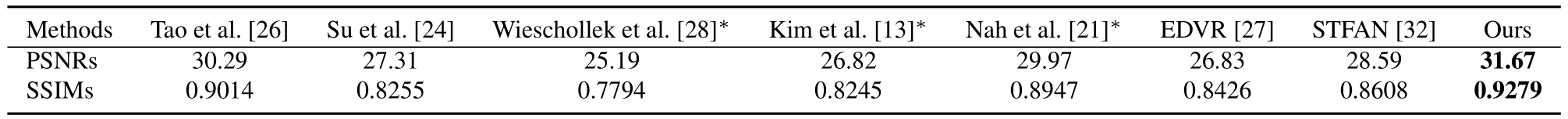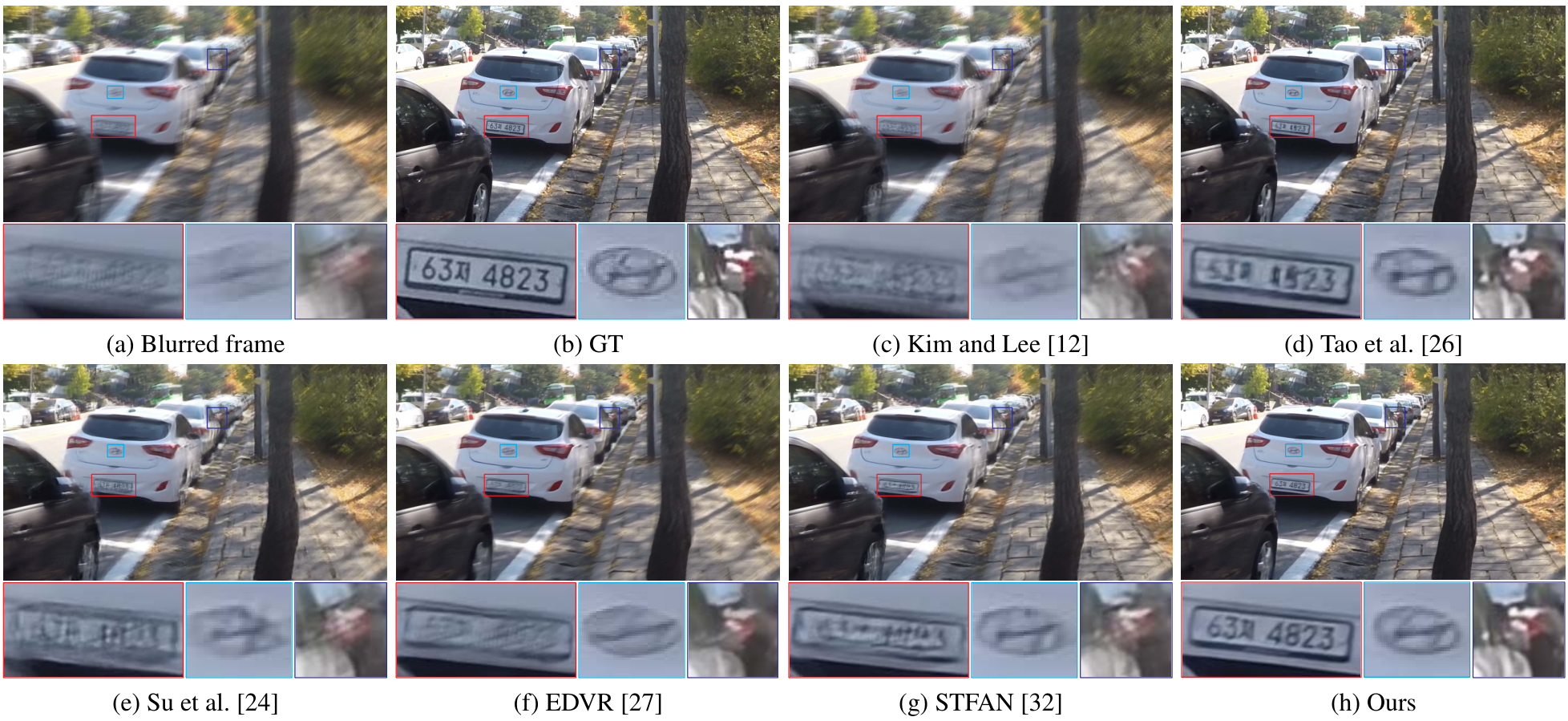### 4.2 真实场景数据集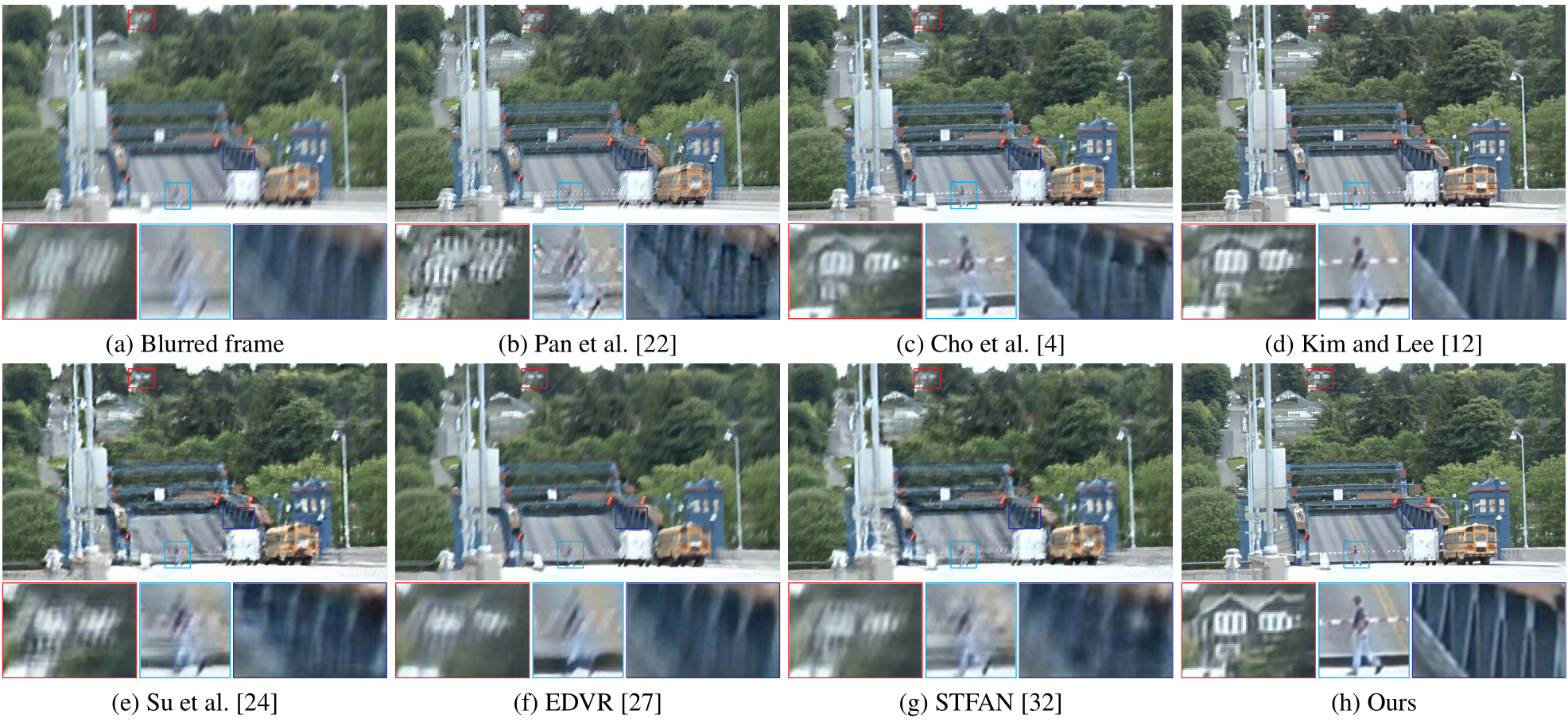## Reference

•  Tae Hyun Kim and Kyoung Mu Lee. Generalized video deblurring for dynamic scenes. In CVPR, pages 5426–5434, 2015.
•  Shangchen Zhou, Jiawei Zhang, Jinshan Pan, Haozhe Xie, Wangmeng Zuo, and Jimmy Ren. Spatio-temporal filter adaptive network for video deblurring. In ICCV, pages 2482–2491, 2019
•  Xintao Wang, Kelvin C.K. Chan, Ke Yu, Chao Dong, and Chen Change Loy. EDVR: Video restoration with enhanced deformable convolutional networks. In CVPR Workshops, 2019.
•  Shuochen Su, Mauricio Delbracio, Jue Wang, Guillermo Sapiro, Wolfgang Heidrich, and Oliver Wang. Deep video deblurring for hand-held cameras. In CVPR, pages 237–246, 2017.
•  Seungjun Nah, Tae Hyun Kim, and Kyoung Mu Lee. Deep multi-scale convolutional neural network for dynamic scene deblurring. In CVPR, pages 257–265, 2017.
•  Sunghyun Cho, Jue Wang, and Seungyong Lee. Video deblurring for hand-held cameras using patch-based synthesis. ACM TOG, 31(4):64:1–64:9, 2012.